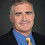## Thursday, November 01, 2018

### P = NP Need Not Give a SAT Algorithm

In Bill's post earlier this week, he asks if there is a fixed algorithm such that if P = NP, this algorithm will correctly compute satisfiability on all inputs. While I believe this statement is true because P ≠ NP, I would like to argue we won't prove it anytime soon.

Bill links to a TCS Stack Exchange answer by Marzio De Biasi giving a fixed algorithm that would get SAT correct except on a finite number of inputs if P = NP. Here is Marzio's algorithm.

Input: x   (boolean formula)
Find the minimum i such that
1) |M_i| < log(log(|x|))  [ M_1,M_2,... is a standard fixed TM enumeration]
2) and  M_i solves SAT correctly
on all formulas |y| < log(log(|x|))
halting in no more than |y|^|M_i| steps
[ checkable in polynomial time w.r.t. |x| ]
if such i exists simulate M_i on input x
until it stops and accept/reject according to its output
or until it reaches 2^|x| steps and in this case reject;
if such i doesn't exist loop for 2^|x| steps and reject.

This proof relativizes: There is a fixed relativizing Turing machine M such that for all A, if PA = NPA then L(MA) runs in polynomial time and differs from SATA on only a finite number of strings. SATA is a relativizable form of SAT with a built in relations that answer whether a sequence of variables encode an string in A. SATA is NPA-complete for all A.

The following shows any proof that a fixed algorithm can compute all of SAT correctly if P = NP cannot relativize.

Theorem: For every deterministic Turing machine M, there is an A such that PA = NPA and either Mdoes not compute SATA correctly on all inputs or Mtakes at least exponential time.

Proof:

Define B = {0x | x in TQBF}. TQBF is the PSPACE-complete problem containing all the true quantified Boolean formula. PTQBF = PSPACE = NPTQBF and thus PB = NPB.

Let φn be the formula that is true if there exists a string z of length n such that 1z is in A. Let n be the smallest n such that MBn) takes less than 2n computational steps. If no such n exists let A = B and we are done.

If MBn) accepts we are done by letting A=B since B has no string beginning with 1.

If MBn) rejects and uses less than 2n steps there must be some z such that MBn) did not query 1z. Let A = B ∪ {1z}.

MAn) still rejects but now φn is in SATA. Also PA = NPA since adding a single string to an oracle won't affect whether two classes collapse.

1.I don't get this Theorem. Wouldn't this imply that there's no M that can compute SAT in polynomial time? (If M never queries the oracle.)

In the proof, isn't the definition of \varphi_n and A circular?

Finally, have you seen Marzio's comments to Bill's post?

1.It's an order of quantifiers. If P^A = NP^A there is an polytime M such that L(M^A) = SAT^A, but my proof shows that M has to depend on A.

I don't see any circularity in the definitions. I'll leave it to Marzio to say how this proof connects with what he commented on Bill's post.

2.OK, after the update of the claim to SAT^A I can understand.

2.Nice!!!

Typo in the theorem definition? "... does not compute ***SAT^A*** correctly on all inputs "

1.Thanks. Fixed the typo.

2.In a comment to Bill's post I said that proving that for a particular A

P=NP -> (polytime (A) AND A=SAT)

Is (logically) equivalent to

P <>NP OR (polytime (A) AND A=SAT)

But polytime (A) AND A=SAT implies P=NP

So proving the above implication is equivalent to solve P=?NP (and clearly such a proof cannot relativize). Is it correct?

3.Not quite. Consider the following statement (true by Levin's construction). There is an algorithm A such that
Factoring is in P -> A in polytime and A solves factoring

As above you have
Factoring not in P OR A in polytime and A solves factoring
and
A in polytime and A solves factoring -> Factoring is in P

But this most certainly does not settle whether factoring is in P.

4.Yate! ( ... yet another trivial error :-)

3.I've posed this somewhat related question: https://cstheory.stackexchange.com/questions/41832/is-there-an-algorithm-that-finds-the-forbidden-minors

4.Also note that any proof that a fixed algorithm can compute all of SAT correctly if P = NP, would imply that P != NP, if true, would be provable in Peano Arithmetic...

1.You need that statement provable in PA, otherwise the argument doesn't work I think.

5.This is somewhat off topic, but if P=NP would that mean that even though cryptography would be very limited, we could still have really good steganography? If P=NP, then machine learning could build very good models of cover texts (i.e. color images, video) that are presumably NP-hard. Does it follow that we could build stego systems that are secure against steganalysis?